Support the Monkey! Tell All your Friends and TeachersHome MonkeyNotes Printable Notes Digital Library Study Guides Study Smart Parents Tips College Planning Test Prep Fun Zone Help / FAQ How to Cite Request a New Title

8.13 Estimation

We have studied so far how to test the hypothesis about Mean or Population proportion from a given sample. Now we are going to determine the Mean or proportion of the population. This is known as estimation. The following types of estimations are possible:

(1) Point estimate and (2) Interval estimate.

Point Estimation: i) The best estimate of population mean ( m ) is the sample mean ( x ) and ii) The best estimate of population proportion (P) is the sample proportion (P').

For example i) If the average weight of 50 students of a school of 500 students is found to be 50 kg. We estimate that the average weight of all 500 students is also 50 kg.

ii) We are given a sample of 100 workers from a population of 1000 workers. If 53% smoke then we estimate that the proportion of smokers in the given population is also 53%.

Interval Estimation: 1) Population mean :

Consider | z | < 1.96 i.e.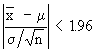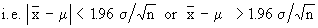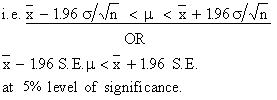This gives us the interval in which we estimate x to lie with 95% confidence. They are called confidence or fiducial limits.

1)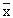± 1.96 S.E. at 95% confidence level.

2)± 2.58 S.E. at 99% confidence level.

3)± 3 S.E. at 99.73% confidence level (which is almost      certainly).

Note : S. E. = Standard Error =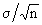However, if population S.D. ( s ) is not known then replace s by s.d. of the sample.Your browser does not support the IFRAME tag.

Example A random sample of 100 girls of 8 graders of a school gave the following results:

Mean weight = 60 kgs. S.D. = 5 kgs. Find the limits within which the mean weight of the school (for girls only) almost certainly lies.

Solution: 99.73% confidence limits of mean are x ± 3 S.E.

Now S.E. =Therefore the limits are 60 - 3 ´ 0.5 = 58.5 kgs and

60 + 3 ´ 0.05 = 61.5 kgs

Example The mean height obtained from a random sample of size 100 is 64 inches. The standard deviation of the distribution of height of the population is known to be 3 inches. Test the statement that the mean height of the population is 67 inches at 5% level of significance. Also set up 99% limits of the mean heights of the population.

Solution: The null hypothesis is that the mean height of the population is 67 inches.

In notation : Ho : m = 67 inches and Ha : m ± 67 inches

Given that m = 67, x = 64 and n = 100 with s = 3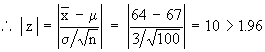Therefore, Ho is rejected at 5% level of significance. Hence mean height of the population could not be 67 inches.

99% probable limits of the mean of population is given by± 2.58 S.E.

Now S.E. =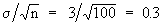Therefore, we have 64 ± 2.58 (0.3) inches .

= 64 - 2.58 (0.3) = 63.2 inches to 64 + 2.58 (0.3) = 64.8 inches.

Index

8.1 Population
8.2 Sample
8.3 Parameters and Statistic
8.4 Sampling Distribution
8.5 Sampling Error
8.6 Central Limit Theorem
8.7 Critical Region
8.8 Testing of Hypothesis
8.9 Errors in Tesitng of Hypothesis
8.10 Power o a Hypothesis Test
8.11 Sampling of Variables
8.12 Sampling of Attributes
8.13 Estimation
8.14 Testing the Difference Between Means
8.15 Test for Difference Between Proportions
8.16 Two Tailed and one Tailed Tests
8.17 Test of Significance for Small Samples
8.18 Students t-distribution
8.19 Distribution of 't' for Comparison of Two Samples Means Independent Samples
8.20 Testing Difference Between Mens of Two Samples Dependent Samples or Matched Paired Observations
8.21 Chi-Square
8.22 Sampling Theory of Correlation
8.23 Sampling Theory of Regression

Chapter 1Search: All Products Books Popular Music Classical Music Video DVD Toys & Games Electronics Software Tools & Hardware Outdoor Living Kitchen & Housewares Camera & Photo Cell Phones Keywords: